# Physics reminder: ohmic and dielectric losses

## Ohmic losses

The flowing of an electric current in a conductor involves energy losses in the form of heat. These losses are called the ohmic losses or losses by Joule effect.

## Dielectric losses

When an AC electric field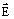is applied to a real dielectric medium, a shift phase appears between the time variations of the electric flux densityand the electric field intensity.

The expl anati on at microscopic scale is the following: the interactions between the molecules tend “to slow down” the rotation of their corresponding dipoles that are trying to align themselves to the field. Thus, the time variation of the polarization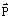is delayed with respect to the electric field intensity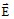. The relation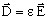valid in a linear medium is no longer applicable for a real quantity ε.

In order to take into account the shift-phase between the electric flux density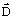and the electric field intensityin harmonic time variation fields, a complex permittivity ε = ε'- j ε " is used.

In a time harmonic electric field:E(t) = E0ejωt

The electric flux density has the expression: D(t) = εE0ejωt = (ε'- jε") E0ejωt

An AC electric field in a dielectric material generates an active power called dielectric hysteresis power losses.

The dielectric hysteresis losses phenomenon is characterized by the quantity: tan δh = ε"/ ε'

where δh is called the dielectric hysteresis losses angle.

## Total losses

An AC electric field in a real material (dielectric and conductor) generates an active power, which is the sum between the ohmic losses and the dielectric losses.

The combination of ohmic and dielectric losses in the real materials is characterized by the quantity:tan δ = (σ + ωε")/(ωε')

where δ is called the losses angle.